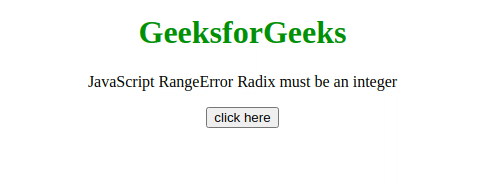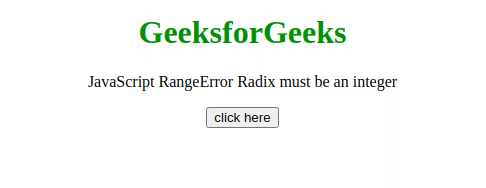Open in App
Not now

# JavaScript RangeError – Radix must be an integer

• Last Updated : 24 Jan, 2023

This JavaScript exception radix must be an integer at least 2 and no greater than 36 occurs if the radix parameter of the Number.prototype.toString() or the BigInt.prototype.toString() method is passed and is not in range between 2 and 36.

Output message:

```RangeError: invalid argument (Edge)
RangeError: radix must be an integer at least 2
and no greater than 36 (Firefox)
RangeError: toString() radix argument must be
between 2 and 36 (Chrome)```

Error Type:

`RangeError`

Cause of the error: When the optional radix parameter of the Number.prototype.toString() or the BigInt.prototype.toString() method is specified and not between 2 and 36, A radix that is greater than 10 starts using alphabet characters as digits which can not be larger than 36(Because alphabet has only 26 letters).

Example 1: In this example, the parameter passed is 2, So the error has not occurred.

## HTML

 `<``body` `style``=``"text-align: center;"``>``    ``<``h1` `style``=``"color: green;"``>``        ``GeeksforGeeks``    ```` ` `    ``<``p``>``        ``JavaScript RangeError``        ``Radix must be an integer``    ```` ` `    ``<``button` `onclick``=``"Geeks();"``>``        ``click here``    ````    ``<``p` `id``=``"GFG_DOWN"``>`` ` `    ``<``script``>``        ``var el_down = document.getElementById("GFG_DOWN");``        ``function Geeks() {``            ``try {``                ``(42).toString(2);``                ``el_down.innerHTML = ``                  ``"'Radix must be an" + ``                  ``" integer' error has not occurred";``            ``} catch (e) {``                ``el_down.innerHTML = ``                  ``"'Radix must be an" + ``                  ``" integer' error has occurred";``            ``}``        ``}``    `````

Output:Example 2:  In this example, the parameter passed is 0, So the error has occurred.

## HTML

 `<``body` `style``=``"text-align: center;"``>``    ``<``h1` `style``=``"color: green;"``>``        ``GeeksforGeeks``    ```` ` `    ``<``p``>``        ``JavaScript RangeError``        ``Radix must be an integer``    ```` ` `    ``<``button` `onclick``=``"Geeks();"``>``        ``click here``    ```` ` `    ``<``p` `id``=``"GFG_DOWN"``>`` ` `    ``<``script``>``        ``var el_down = document.getElementById("GFG_DOWN");``        ``function Geeks() {``            ``try {``                ``(42).toString(0);``                ``el_down.innerHTML = ``                  ``"'Radix must be an" + ``                  ``" integer' error has not occurred";``            ``} catch (e) {``                ``el_down.innerHTML = ``                  ``"'Radix must be an" +``                  ``" integer' error has occurred";``            ``}``        ``}``    `````

Output:My Personal Notes arrow_drop_up